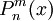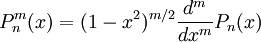# Associated Legendre functions

(diff) ← Older revision | Latest revision (diff) | Newer revision → (diff)
The associated Legendre functions$P^m_n(x)$ are most conveniently defined in terms of derivatives of the Legendre polynomials:$P^m_n(x)= (1-x^2)^{m/2} \frac{d^m}{dx^m} P_n(x)$